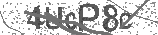﻿ How To Graph Equations in Algebra | Safe Videos for Kids
Welcome

# How To Graph Equations in Algebra

Thanks! Share it with your friends!

URL

You disliked this video. Thanks for the feedback!

Sorry, only registred users can create playlists.URLChannel: The Organic Chemistry Tutor
Categories: Algebra   |   MathFind Related Videos  added
23 Views## Description

This algebra video tutorial explains how to graph equations such as linear equations in slope intercept form & standard form as well as quadratic functions using transformations, factoring, & setting up a data table.

Algebra Textbooks:
https://amzn.to/3cetPpC

My Website: https://www.video-tutor.net
Patreon: https://www.patreon.com/MathScienceTutor
Amazon Store: https://www.amazon.com/shop/theorganicchemistrytutor

Here is a list of other useful videos:
1. How To Graph Quadratic Functions In Vertex Form:

2. How To Graph Quadratic Functions In Standard Form:

3. How To Graph Quadratic Equations Using a Data Table:

4. How To Graph Radical Functions:

5. How To Graph Absolute Value Functions:

6. How To Graph Piecewise Functions:

7. How To Graph Rational Functions:

8. How To Graph Exponential Functions:

9. How To Graph Logarithmic Functions:Be the first to comment